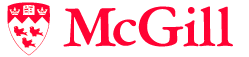# COMP 360: Algorithm Design

## Course Outline

• Introduction
• Stable Matching.
• Network Flows
• Max Flow Min Cut Theorem;
• Algorithms for Max Flow;
• Applications: Bipartite Matching, Vertex Cover etc.
• Linear Programming
• (Weak and Strong) Duality, Complementary Slackness;
• Algorithms for solving LPs (Simplex, Ellipsoid);
• Application of LPs.
• NP and NP-completeness
• Reductions;
• NP-completeness and proof of Cook’s Theorem.
• Heuristics
• Backtracking: Hamiltonian Cycle, Satisfiability ;
• Branch and Bound ;
• Local Search.
• Approximation Algorithms
• Vertex Cover;
• Traveling Salesman.
• Randomized Algorithms
• Minimum Cut;
• Markov Chain Monte Carlo (MCMC), Simulated Annealing.
• Other topics we may cover
• Online Algorithms;
• Fixed-Parameter Tractability;
• Complexity: PSPACE, EXP.